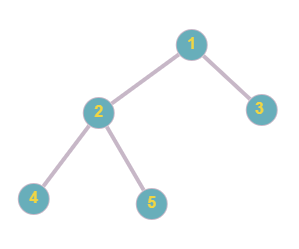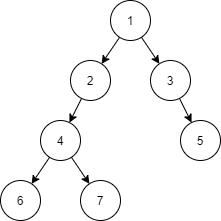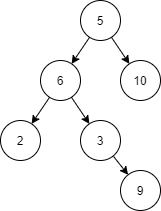2

# Preorder Traversal

Difficulty: EASYContributed By
Srejan Kumar Bera
Avg. time to solve
15 min
Success Rate
85%

Problem Statement

#### You are given the root node of a binary tree consisting of ‘N’ nodes. Your task is to return its preorder traversal. The preorder traversal of a binary tree is defined as a process of traversing each node in the following manner-:

``````1- Visit the root node.
2- Traverse all nodes in the left subtree of the root node.
3- Traverse all the nodes in the right subtree of the root node.
``````
##### For Example:
``````For the given tree below,
Preorder traversal for the given tree will be [1, 2, 4, 5, 3]. Hence, the answer is [1, 2, 4, 5, 3].
``````##### Example:
``````Elements are in the level order form. The input consists of values of nodes separated by a single space in a single line. In case a node is null, we take -1 in its place.

For example, the input for the tree depicted in the below image would be :
````````````1
2 3
4 -1 5 6
-1 7 -1 -1 -1 -1
-1 -1

Explanation :
Level 1 :
The root node of the tree is 1

Level 2 :
Left child of 1 = 2
Right child of 1 = 3

Level 3 :
Left child of 2 = 4
Right child of 2 = null (-1)
Left child of 3 = 5
Right child of 3 = 6

Level 4 :
Left child of 4 = null (-1)
Right child of 4 = 7
Left child of 5 = null (-1)
Right child of 5 = null (-1)
Left child of 6 = null (-1)
Right child of 6 = null (-1)

Level 5 :
Left child of 7 = null (-1)
Right child of 7 = null (-1)

The first not-null node (of the previous level) is treated as the parent of the first two nodes of the current level.

The second not-null node (of the previous level) is treated as the parent node for the next two nodes of the current level and so on.

The input ends when all nodes at the last level are null (-1).
``````
##### Note :
``````The above format was just to provide clarity on how the input is formed for a given tree.

The sequence will be put together in a single line separated by a single space. Hence, for the above-depicted tree, the input will be given as:

1 2 3 4 -1 5 6 -1 7 -1 -1 -1 -1 -1 -1
``````
##### Input Format:
``````The first line of the input contains an integer, 'T,’ denoting the number of test cases.

The first line of each test case contains the elements of the tree in the level order form separated by a single space. If any node does not have a left or right child, take -1 in its place. Refer to the example for further clarification.
``````
##### Output Format:
``````For each test case, print a single line of space-separated integers denoting the preorder traversal of the given binary tree.

Print the output of each test case in a separate line.
``````
##### Note:
``````You do not need to print anything. It has already been taken care of. Just implement the given function.
``````
##### Constraints:
``````1 <= T <= 10
1 <= N <= 10^4
1 <= nodeVal <=10^9

Time limit: 1 sec
``````
##### Sample Input 1:
``````2
1 2 3 4 -1 -1 5 6 7 -1 -1 -1 -1 -1 -1
5 6 10 2 3 -1 -1 -1 -1 -1 9 -1 -1
``````
##### Sample Output 1:
``````1 2 4 6 7 3 5
5 6 2 3 9 10
``````
##### Explanation:
``````For the first test case, the given tree is:
````````````Preorder traversal will be : [1, 2, 4, 6, 7, 3, 5]. Hence, the answer is [1, 2, 4, 6, 7, 3, 5].

For the second test case, the given tree is
````````````Preorder traversal will be : [5, 6, 2, 3, 9, 10]. Hence, the answer is  [5, 6, 2, 3, 9, 10].
``````
##### Sample Input 2:
``````2
1 2 3 4 -1 7 8 5 -1 9 -1 -1 -1 6 -1 -1 -1 -1 -1
10 20 11 -1 -1 -1 -1
``````
##### Sample Output 2:
``````1 2 4 5 6 3 7 9 8
10 20 11
``````Console